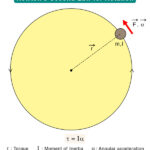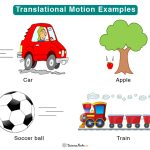Home / Physics / Magnetic Energy

# Magnetic Energy

Magnetic energy is the energy associated with a magnetic field. Since electric currents generate a magnetic field, magnetic energy is due to electric charges in motion.

Magnetic fields are generated by permanent magnets, electromagnets, and changing electric fields. Energy is stored in these magnetic materials to perform work and is different for different materials. Since it is stored energy, magnetic energy is a form of potential energy.

Scottish mathematician and scientist James Clerk Maxwell are credited with having discovered magnetic energy during his pioneering work on electromagnetism in 1865.

## How is Magnetic Energy Calculated

The magnetic energy is determined by calculating the magnetic energy density. It is denoted by the symbol ρm and is given by the following formula.

$\rho_m = \tfrac{1}{2} BH = \tfrac{1}{2}\mu_oH^2 = \frac{1}{2}\frac{B^2}{\mu_o}$

The total energy, E, is the integral of ρm over a given volume.

$E = \int \rho_m dV$

Which gives the following expression:

$E = \tfrac{1}{2}LI^2$

Where,

H: Magnetic field strength

B: Magnetic field

μo: Permeability of free space

I: Current

Unit: Joules or J

## Examples and Uses

• A generator uses magnetic energy to generate electricity
• An electrical transformer uses magnetic energy to step up and down the voltage
• Maglev train operates on magnetic energy
• Some machines hold parts together by using magnetic energy
• Refrigerators, headphones, and hard-disk run on magnetic energy

## Magnetic Energy and Electric Energy

Maxwell found that two primary forms of energy, electric and magnetic energy, are not significantly different. They are closely associated. Electrical current results in its magnetic field, and changing magnetic field creates current. When magnetic energy is naturally combined with electric energy, it is called electromagnetic energy.

## FAQs

Q.1. Why aren’t magnets used for energy?

Ans. Magnets are not used as energy because they do not inherently possess any energy. For example, in a generator, we do not get energy from the magnetic field. The energy going into the electrical current comes from the energy required to spin the coil between the two magnets.

Q.2. How can magnets cause objects to have kinetic energy?

Ans. Magnets can cause objects to have kinetic energy due to magnetic force. The force pulls ferromagnetic substances, like nails and paper clips. In this process, potential energy is converted into kinetic energy.

Article was last reviewed on Friday, July 22, 2022

### Related articlesNewton’s Second Law for RotationRotational MotionTranslational MotionChemical Energy